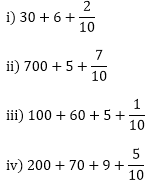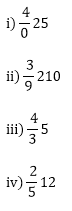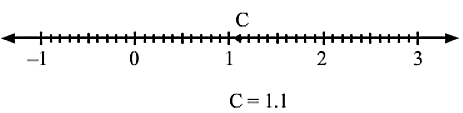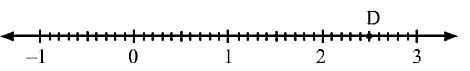×#### Thank you for registering.

One of our academic counsellors will contact you within 1 working day.

Click to Chat

1800-1023-196

+91-120-4616500

CART 0

• 0

MY CART (5)

Use Coupon: CART20 and get 20% off on all online Study Material

ITEM
DETAILS
MRP
DISCOUNT
FINAL PRICE
Total Price: Rs.

There are no items in this cart.
Continue Shopping• Complete JEE Main/Advanced Course and Test Series
• OFFERED PRICE: Rs. 15,900
• View Details

```Chapter 7: Decimals – Exercise 7.2

Question: 1

i) Three tenths
ii) Two ones and five tenths
iii) Thirty and one tenths
iv) Twenty two and six tenths
v) One hundred, two ones and three tenths

Solution:

i) 3/10 = 0.3
ii) 2+ 5/10 = 2.5
iii) 30 +1/10 = 30.1
iv) 22+ 6/10 = 22.6
v) 100 + 2 + 3/10 = 102.3

Question: 2Solution:

i) We have 3 tens, 6 ones and 2 tenths. Therefore, the decimal is 36.2
ii) We have 7 hundreds, 5 ones and 7 tenths. Therefore the decimal is 705.7
iii) We have 2 hundreds, 6 tens, 5 ones and 1 tenths. Therefore the decimal is 265.1
iv) We have 2 hundreds, 7 tens, 9 ones and 5 tenths. Therefore, the decimal is 279.5

Question: 3

i) 22/10
ii) 3/2
iii) 2/5

Solution:

i) Since the denominator is ten, the decimal is 2.2
ii) Making the denominator 10, we have 3/2

3(52)(5) = 1510 = 1.5
iii)  Making the denominator 10, we have 2/5

2(25)(2) = 410 = 0.4

Question: 4Solution:

i) To write in decimal, we need to make the denominator 10 by multiplying it by a number. But, to maintain the value of the fraction, we should also multiply the numerator by the same number. Thus, we get

= 40 + 2(25)(2) = 40 + 410 = 40.4

ii)  39210 = 39 +210

Here, the denominator is 10 .

Therefore, the decimal is 39.2

iii) 435 = 4 + 35

To write in decimal, we need the denominator by 10 by multiplying it by a number. but, to maintain the value of the fraction, we should also multiply the numerator by the same number. Thus we get,

= 4 + 3(3)(25)(2)

= 4 + 610 = 4.6

iv) 2512 = 25 +12

To write in decimal, we need to make the denominator 10 by multiplying it by a number. But, to maintain the value of the fraction, we should also multiply the numerator by the same number. Thus, we get

= 25 + 1(52)(5)

= 25 + 510 = 25.5

Question: 5

i) 3.8

ii) 21.2

iii) 6.4

iv) 1

Solution:

i) 3.8

= 3 + 8 tenths

= 3 + 810

= 3(1010) + 810 = 3010 + 810 = 3810 = 195

ii) 21.2

=21+2 tenths

= 21+210= 21(1010) + 210 = 21010 + 210 = 21210 = 1065

iii) 6.4

= 6 + 4 tenths

= 6 + 410

= 6(1010) +410 = 6010 + 410 = 325

iv) 1

Since the only number after the decimal is 0, the fraction is 1

Question: 6

Represent the following number on the number line.

i) 0.2
ii) 1.9
iii) 1.1
iv) 2.5

Solution:

i)ii)iii)iv)Question: 7

i) 0.8 is between the two whole numbers 0 and 1

ii) 5.1 is between the two whole number 5 and 6.

iii) 2.6 is between 2 and 3

iv) 6.4 is between 6 and 7

Solution:

i) As 0.8 is 8 units from 0 and 2 units from 1, it is nearer to 1
ii) As 5.1 is 1 unit from 5 and 9 units from 6, it is nearer to 5
iii) As 2.6 is 6 units from 2 and 4 units from 3, it is nearer to 3
iv)  As 6.4 is 4 units from 6 and 6 units from 7, it is nearer to 6

9.0 is itself a whole number, that is 9

4.9 is between 4 and 5

As 4.9 is 9 units from 4 and 1 unit from 5, it is nearer to 5

Question: 8

Write the decimal number represented by the points on the given number line A, B, C, DSolution:

A) 0.8, since A is at the eighth place between 0 and 1

B) 1.3, since B is at the third place between 1 and 2

C) 1.9, since C is at the ninth place between 1 and 2

D) 2.6, since D is at the sixth place between 2 and 3

Disclaimer: the image given in the book is not consistent; as the number of periods between 0 and 1 is ten but the number of periods between 1 and 2 are seven. So, ignoring the position of the given numbers 1, 2 and 3. it has been assumed that there are ten periods between every two consecutive numbers starting from the first point taken as zero.

```### Course Features

• 728 Video Lectures
• Revision Notes
• Previous Year Papers
• Mind Map
• Study Planner
• NCERT Solutions
• Discussion Forum
• Test paper with Video Solution